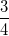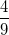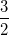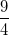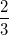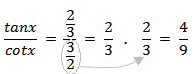Trigonometry : Trigonometric Applications

Example Questions

Example Question #1 : Trigonometric Applications

Given a right triangle where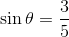, find the missing side.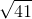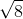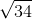Explanation:

Since the triangle in question is a right triangle we can use the Pythagorean Theorem. First, we must determine which sides we are given. Since the function we are given is sine, we know that we are given the opposite side and the hypotenuse. Therefore, setting up the equation:Where,andare given.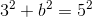Solving the above equation: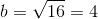We toss out the negative solution since the length of a side must be positive.

Example Question #2 : Right Triangles

Given a right triangle where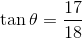, find the missing side.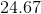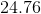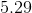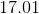Explanation:

Since the triangle in question is a right triangle we can use the Pythagorean Theorem. First, we must determine which sides we are give. Since the function we are given is tangent, we know that we are given the opposite and adjacent sides. Therefore, setting up the equation:Where,andare given.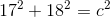Solving the above equation: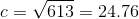We toss out the negative solution since the length of a side must be positive.

Example Question #2 : Trigonometric Applications

Given a right triangle where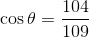, find the missing side.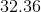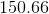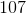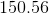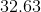Explanation:

Since the triangle in question is a right triangle we can use the Pythagorean Theorem. First, we must determine which sides we are given. Since the function we are given is cosine, we know that we are given the adjacent side and hypotenuse. Therefore, setting up the equation:Where,andare given.Solving the above equation: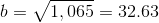We toss out the negative solution since the length of a side must be positive.

Example Question #4 : Right Triangles

Given the accompanying triangle whereand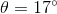, determine the length of the hypotenuse.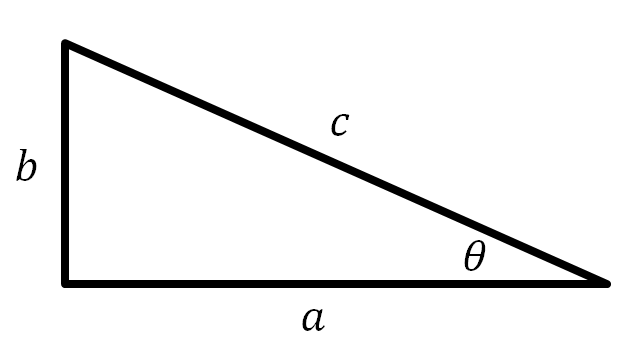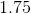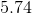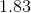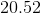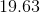Explanation:

We are given the opposite side, with respect to the angle, along with the angle. Therefore, we utilize the sine function to determine the length of the hypotenuse: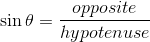Substituting the given values: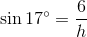Cross multiplying: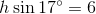Solving for: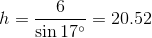Example Question #3 : Trigonometric Applications

Given the accompanying right triangle where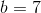and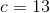, determine the measure of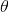to the nearest degree.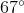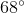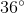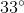Explanation:

We are given two sides of the right triangle, namely the hypotenuse and the opposite side of the angle. Therefore, we simply use the sine function to determine the angle: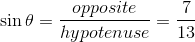In order to isolate the angle we must apply the inverse sine function to both sides: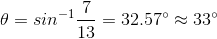Example Question #6 : Right Triangles

Given the accompanying right triangle where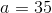and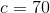, determine the measure ofto the nearest degree.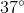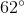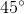Explanation:

We are given two sides of the right triangle, namely the hypotenuse and the adjacent side of the angle. Therefore, we simply use the cosine function to determine the angle: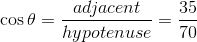In order to isolate the angle we must apply the inverse cosine function to both sides: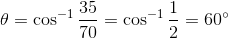Example Question #7 : Right Triangles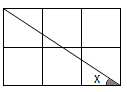All the squares are equal, and there are 6 squares in the figure.

What is the value of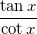?